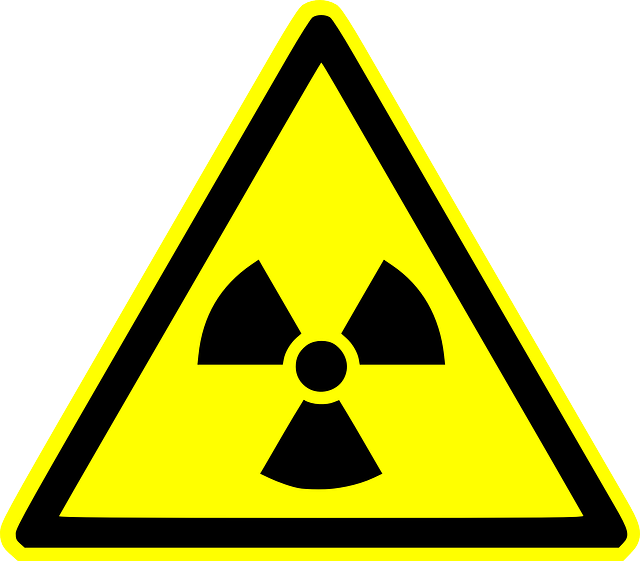# Radioactivity: What’s tested in O Level PhysicsA new topic, radioactivity will be tested in O Level Physics starting from 2024 (this means 2023 secondary 3 students will learn this topic for O Level, probably in secondary 4). In this topic, students are tested on the composition of atoms, radioactivity decay (alpha, beta and gamma particles), as well as their applications and dangers.

The first part of radioactivity tests students on their understanding of atomic structure, and then move on to talk about alpha, beta and gamma radiation. Understanding the radioactive decay section will help students greatly in the application part.

## What’s tested in Radioactivity for O Level Physics?

Here’s the syllabus for radioactivity of O Level Physics in short. It can be divided into 3 parts:

• Composition of atoms
• Dangers and Use of Radioactivity

## Composition of atoms

For students taking Chemistry, this section should be simply a revision. This section tests students on:

• composition of atoms (i.e. electrons, protons and neutrons), and their charges
• understanding proton number or atomic number and neucleon number or mass number
• being able to interpret the number of protons and neutrons from nuclide notation

In this section students need to know:

• radioactive decay is a random and spontaneous process, and what random and spontaneous means
• the 3 types of radiation: alpha, beta and gamma
• use equations involving nuclide notation to represent changes in the composition of the nucleus when radioactive emissions occur